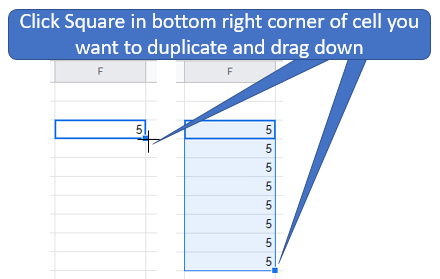# 3: Google Workbook (Sheets)- Data Manipulation

$$\newcommand{\vecs}{\overset { \rightharpoonup} {\mathbf{#1}} }$$ $$\newcommand{\vecd}{\overset{-\!-\!\rightharpoonup}{\vphantom{a}\smash {#1}}}$$$$\newcommand{\id}{\mathrm{id}}$$ $$\newcommand{\Span}{\mathrm{span}}$$ $$\newcommand{\kernel}{\mathrm{null}\,}$$ $$\newcommand{\range}{\mathrm{range}\,}$$ $$\newcommand{\RealPart}{\mathrm{Re}}$$ $$\newcommand{\ImaginaryPart}{\mathrm{Im}}$$ $$\newcommand{\Argument}{\mathrm{Arg}}$$ $$\newcommand{\norm}{\| #1 \|}$$ $$\newcommand{\inner}{\langle #1, #2 \rangle}$$ $$\newcommand{\Span}{\mathrm{span}}$$ $$\newcommand{\id}{\mathrm{id}}$$ $$\newcommand{\Span}{\mathrm{span}}$$ $$\newcommand{\kernel}{\mathrm{null}\,}$$ $$\newcommand{\range}{\mathrm{range}\,}$$ $$\newcommand{\RealPart}{\mathrm{Re}}$$ $$\newcommand{\ImaginaryPart}{\mathrm{Im}}$$ $$\newcommand{\Argument}{\mathrm{Arg}}$$ $$\newcommand{\norm}{\| #1 \|}$$ $$\newcommand{\inner}{\langle #1, #2 \rangle}$$ $$\newcommand{\Span}{\mathrm{span}}$$$$\newcommand{\AA}{\unicode[.8,0]{x212B}}$$

### Basic Data Maniuplation

Copying ValuesFigure $$\PageIndex{1}$$: Copy and Paste Caption here. (Copyright; author via source)

### Formulas

#### Basics

Sheet Skills

Formulas Basics: Use formulas, reference cells

Instructions

Google Sheets has built in tools to be able to do many things in sheets called formulas. There is a full list of formulas by google sheets. We're going to start off with some of the basics then later on go more in depth.

1. To start using a formula first select the cell you want to use
2. Type an equals sign = to start using a formula in the cell you want to use
3. Start to type the formula you wish to use and select it from the drop down menu
4. You can type a number or use a cell reference, you can either type the reference (For example A2) or click the cell you are wanting to reference
5. Finally press enter

#### Simple Mathematical Functions

Sheet Skills Video

Simple Mathematical Functions: Addition, Subtraction, Multiplication, Division, Exponents, Parentheses

Instructions

You can do simple calculations using formulas. Start all formulas by typing =

Subtraction -

Multiplication *

Division /

Exponent ^

Parentheses ()

So for example if we can use the sheet to calculate the gravitational force between two objects

Video $$\PageIndex{1}$$: 1:40 Video example of how to do basic calculations in sheets ()Figure $$\PageIndex{1}$$: Calculating the gravitational force of two objects. Formula used : =D2*((A2*B2)/C2^2) (Poirot)

#### Logarithms

Sheet Skills Video

Logarithms: Log and LN

Instructions

Logarithms are used in many different calculations. There is an overview on logarithms in Chapter ? that explains how logs work. In this section we are going to focus on how to use them in sheets.

LOG10

Returns the the logarithm of a number, base 10. =LOG10(value)

This is probably what you will use most often when talking about logarithms. This function will give the logarithm of a number using base 10.

LOG

Returns the the logarithm of a number given a base. =LOG(value, [base])

This is used less often but can be used to return a logarithm using any other base. This function will assume base 10 unless told otherwise

LN

Returns the the logarithm of a number, base e (Euler's number). =LN(value)

This function gives the natural log of a number. You can also do the natural log using LOG by entering the base as "EXP(1)"

Video $$\PageIndex{2}$$: 2:11 Video tutorial on log, ln and 1/T. (https://youtu.be/q-gALwjPj9I)

*Note if you are doing the Graphing lab you are using Ln(s) vs T not 1/T

This page titled 3: Google Workbook (Sheets)- Data Manipulation is shared under a not declared license and was authored, remixed, and/or curated by Robert Belford.